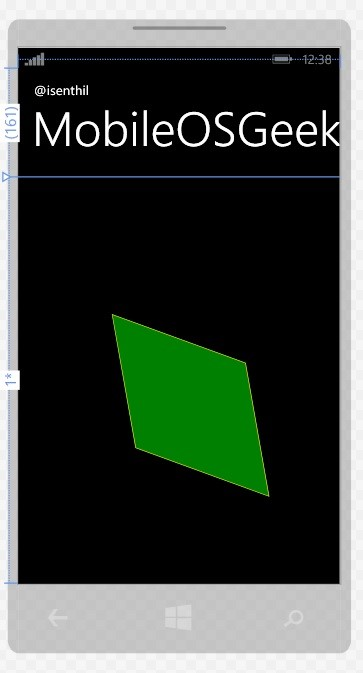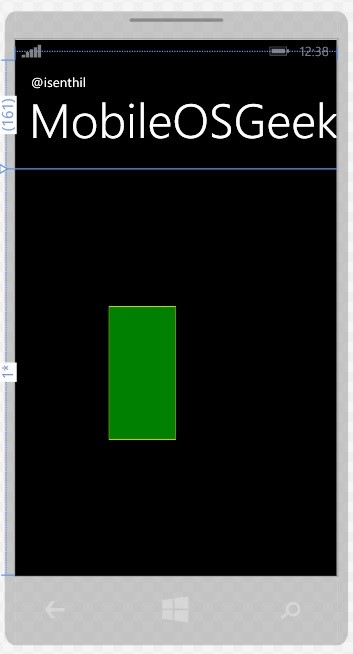Different Transforms in XAML and Windows Phone

This blog post will introduce and demonstrate the different transforms in XAML that can be used in a Windows Phone page.

There are 5 different types of transforms that can be applied for an XAML element in the Windows Phone page. These includes

• Rotate Transform
• Translate Transform
• Scale Transform
• Skew Transform
• Matrix Transform

Different Transforms in Windows Phone

1. Rotate Transform

The RotateTransform lets the developers to rotate the points of the element around the original point. The value by which the element to be rotated is specified by the angle property which accept numeric (both positive and negative).

Below is a sample code snippet demonstrating the usage of the RotateTransform to rotate the Rectangle by -45 degree using the RenderTransform and RotateTransform element.

<Grid x:Name="ContentPanel" Grid.Row="1" Margin="12,0,12,0">

<Rectangle Width="200" Height="200"

Fill="Green" Stroke="Yellow"

Canvas.Left="0" Canvas.Top="50" RenderTransformOrigin="0,0" >

<Rectangle.RenderTransform>

<RotateTransform Angle="-45"/>

</Rectangle.RenderTransform>

</Rectangle>

</Grid>2. Translate Transform

The TranslateTransform is used to move an object by the specified amount of pixels. The object is moved along the specified X and Y axis.

Below is a sample code snippet demonstrating the usage of the TranslateTransform to move the Rectangle by 10px on both X and Y axis.

<Grid x:Name="ContentPanel" Grid.Row="1" Margin="12,0,12,0">

<Rectangle Width="200" Height="200"

Fill="Green" Stroke="Yellow"

Canvas.Left="0" Canvas.Top="50" RenderTransformOrigin="0,0" >

<Rectangle.RenderTransform>

<TranslateTransform X="10" Y="10" />

</Rectangle.RenderTransform>

</Rectangle>

</Grid>3. ScaleTransform

The ScaleTransform is used to multiple the size of an object by the specified scale factor along the X and Y axis.

<Grid x:Name="ContentPanel" Grid.Row="1" Margin="12,0,12,0">

<Rectangle Width="200" Height="200"

Fill="Green" Stroke="Yellow"

Canvas.Left="0" Canvas.Top="50" RenderTransformOrigin="0,0" >

<Rectangle.RenderTransform>

<ScaleTransform ScaleX="0.5" ScaleY="1"/>

</Rectangle.RenderTransform>

</Rectangle>

</Grid>4. SkewTransform

The SkewTransform skews/ bends the borders of the object with the specified angle. Below is a sample code snippet demonstrating the usage of the SkewTransform.

<Grid x:Name="ContentPanel" Grid.Row="1" Margin="12,0,12,0">

<Rectangle Width="200" Height="200"

Fill="Green" Stroke="Yellow"

Canvas.Left="0" Canvas.Top="50" RenderTransformOrigin="0,0" >

<Rectangle.RenderTransform>

<SkewTransform AngleX="10" AngleY="20"/>

</Rectangle.RenderTransform>

</Rectangle>

</Grid>5. MatrixTransform

The last type of transformation is the MatrixTransform. The MatrixTransform is a 3 X 3 matrix and the object is transformed by this.

The MatrixTransform has the properties M11, M12, M21, M22, OffsetX, and OffsetY and all the transforms like Rotate , Scale etc. can be achieved by setting the right values to these properties in the MatrixTransform.

Below is a sample code snippet demonstrating how to achieve the ScaleTransform with the MatrixTransform.

<Grid x:Name="ContentPanel" Grid.Row="1" Margin="12,0,12,0">

<Rectangle Width="200" Height="200"

Fill="Green" Stroke="Yellow"

Canvas.Left="0" Canvas.Top="50" RenderTransformOrigin="0,0" >

<Rectangle.RenderTransform>

<MatrixTransform>

<MatrixTransform.Matrix>

<Matrix M11="0.5" M12="0" OffsetX="0" M21="0" M22="1" OffsetY="0" />

</MatrixTransform.Matrix>

</MatrixTransform>

</Rectangle.RenderTransform>

</Rectangle>

</Grid>This blog post listed out some of the commonly used transforms in XAML and Windows Phone. I will try to cover each one of them in depth in my future blog posts.

One thought on “Different Transforms in XAML and Windows Phone”

1. Thanks a lot dude….